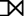# Extended Operators in DBMS

## Extended Operators in DBMS

In this article, we will learn about Extended Operators in DBMS. There are different types of Extended Operators which is explained below in brief.#### Relational Algebra in DBMS

• `Relational algebra` is a widely used procedural query language.
• Relational algebra collects instances of relations as input and gives occurrences of relations as output by using various operations.
• Relational algebra operations are performed recursively on a relation.
• The output of relational algebra operations is a new relation, which can be formed from one or more input relations.

#### Extended Operators in Relational Algebra:

Extended operators in Relational algebra are:

•  Natural Join (⋈)
• Left, Right, Full outer join (⟕, ⟖, ⟗)
• Intersection (∩)

### Natural Join (⋈)

• JOIN operation also allows joining variously related tuples from different relations.
• Natural join can only be performed if there is a common attribute (column) between the relations.
• The name and type of the attribute must be same.

#### Example:

Consider the following two tables:

Table C

 Num Square 2 4 3 9

Table D

 Num Cube 2 8 3 27

```C ⋈ D
```
 Num Square Cube 2 4 8 3 9 27

### Outer Join

• In an outer join, along with tuples that satisfy the matching criteria, we also include some or all tuples that do not match the criteria.
• There are three types of outer joins. They are
• Left Outer Join
• Right Outer Join
• Full Outer Join
• Consider the following 2 Tables :

Table A

 Num Square 2 4 3 9 4 16

Table B

 Num Cube 2 8 3 27 5 125

#### Left Outer Join(AB)

• In the left outer join, the operation allows keeping all tuple in the left relation.
• If there is no matching tuple is found in the right relation, then the attributes of the right relation in the join results are filled with null values.

#### Example:

```AB
```
 Num Square Cube 2 4 8 3 9 27 4 16 –

#### Right Outer Join(AB)

• In the right outer join, the operation allows keeping all tuple in the right relation.
• If there is no matching tuple is found in the left relation, then the attributes of the left relation in the join results are filled with null values.

#### Example:

```AB
```
 Num Square Cube 2 4 4 3 9 9 5 – 125

#### Full Outer Join: ( AB)

In a full outer join, all tuples from both relations are included in the result, irrespective of the matching condition.

```AB
```
 Num Cube Square 2 4 8 3 9 27 4 16 – 5 – 125

### Intersection (∩)

• An intersection is defined by the symbol ∩
• A ∩ B defines a relation consisting of a set of all tuple that is in both A and B. However, A and B must be union-compatible.

#### Example:

Consider 2 tables –

Table A :

 Attribute1 Attribute2 1 1 1 2

Table B :

 Attribute1 Attribute2 1 1 1 3

`A ∩ B`
 Attribute1 Attribute2 1 1